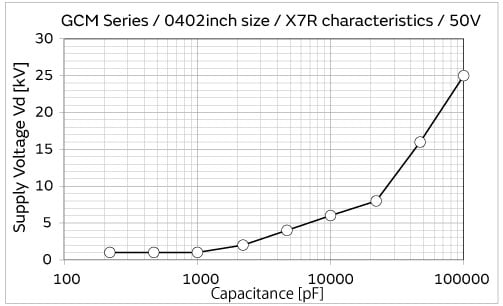Capacitor Guide

# ESD Resistance of Capacitors

The ESD (Electrostatic Discharge) resistance of capacitors is described in the following.

## ESD Resistance Test Method

When static electricity charged to people or equipment is discharged to electronic devices or components, an electromagnetic energy shock is applied; therefore capacitors must have a constant ESD resistance or more.

There are three test methods for ESD resistance: (1) HBM, (2) MM, and (3) CDM as shown in the following, depending on the model that generates the static.

Among these tests, (1) HBM, the most commonly used test method for ESD resistance of capacitors, is described in the following.

 ① HBM  (Human Body Model) ： Test based on the assumption that static is discharged from a human body ② MM  (Machine Model) ： Test based on the assumption that static is discharged from a machine ③ CDM  (Charged Device Model) ： Test based on the assumption that static is discharged from an electrified device
As ESD test standards of HBM, there are the AEC-Q200-002, IEC61000-4-2, etc., and the HBM model constant differs by the standard as shown in the table below.
Standards Charging Capacitor Cd（pF） Discharge Resistor Rd （Ω）
AEC-Q200-002 150 2000
IEC61000-4-2 150 330

The HBM ESD test circuit and discharge current waveform of AEC-Q200-002 is shown in Figure 1 and Figure 2.

 （i） While switch 2 is open, switch 1 is closed, and a high voltage power supply is applied to accumulate electricity in the charging capacitor (Cd). （ii） Switch 1 is open, switch 2 is closed, and the electricity that accumulated in the Cd is applied to the test capacitor (Cx) to perform the test.

※Cx：Test capacitor, Cd: Charging capacitor, Rd: Discharge resistor, Rc: Protective resistance

In AEC-Q200-002, the flow of the ESD test of HBM is shown in Figure 3, and the class classification is shown in Table 1. The test was implemented according to the flow of Figure 3, and the rank of the withstand voltage is classified according to Table 1.

Table 1. HBM ESD Test Flow
Classification Maximum Withstand Voltage
1A Less than 500V (DC)
1B 0.5 kV (DC) or more, Less than 1 kV (DC)
1C 1 kV (DC) or more, Less than 2 kV (DC)
2 2 kV (DC) or more, Less than 4 kV (DC)
3 4 kV (DC) or more, Less than 6 kV (DC)
4 6 kV (DC) or more, Less than 8 kV (DC)
5A 8 kV (DC) or more, Less than 12 kV (AD)
6 25 kV (AD) or more

## Relationship between Capacitance and ESD Resistance of Capacitors

The capacitance of the test capacitor affects the voltage that occurs on both sides of a capacitor.

The following relationship is established between the capacitance (Cx) of the test capacitor, and the voltage (Vx) that occurs on both sides.

While the supply voltage (Vd) and the capacitance of the charging capacitor (Cd) is constant, the voltage (Vx) that occurs on both sides of the test capacitor becomes lower as the capacitance (Cx) of the test capacitor becomes larger.

Therefore, generally, the ESD resistance tends to become higher as the capacitance of the test capacitor becomes larger.

In fact, since the marginal performance of the withstand voltage changes with the differences in the design, such as the type and thickness of the dielectrics, the value does not always match the tendency mentioned above.

## (Reference Data) ESD Resistance of Multilayer Ceramic Capacitors (GCM Series)

The ESD resistance reference data of multilayer ceramic capacitors (GCM series) is shown below.

Applicable Product GCM Series / 0402 inch size / X7R characteristics / 50V
Test Conditions

Charging capacitor = 150pF

Discharge resistance = 2000Ω

No. of applications = 5 times each for positive/negative polarity

No. of samples = 10 samples each

Measuring Device ESD tester (Noise Laboratory Co., Ltd.)
・Tester ... ESS-200AX
・Discharge gun ... Model TC-815D
Condition of Samples Surface is covered with silicon resin to prevent surface leaks
Periphery Environment 22±5 °C, 30 to 60% RH
Acceptance Criteria Insulation resistance:　1MΩFigure 5: High Dielectric Type Capacitor ESD Test Results (Vd = 25 kV is limit of measurement)